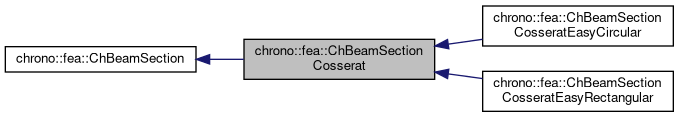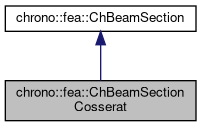chrono::fea::ChBeamSectionCosserat Class Reference

## Description

Base class for properties of beam sections of Cosserat type (with shear too) such as ChElementBeamIGA.

A beam section can be shared between multiple beams. A beam section contains the models for elasticity, inertia, plasticity, damping, etc. This base model expect that you provide at least the elasticity and inertia models, and optionally you can also add a damping model and a plasticity model. This accomodates most of the constitutive models because there are many combinations of the different types of damping models, elasticity models, etc., but if you need some extreme customization, you might also inherit your C++ class from this. On the other side, if you need a more immediate way to create sections, look at the special cases called ChBeamSectionCosseratEasyRectangular and ChBeamSectionCosseratEasyCircular.

#include <ChBeamSectionCosserat.h>

Inheritance diagram for chrono::fea::ChBeamSectionCosserat:[legend]
Collaboration diagram for chrono::fea::ChBeamSectionCosserat:[legend]

## Public Member Functions

ChBeamSectionCosserat (std::shared_ptr< ChInertiaCosserat > minertia, std::shared_ptr< ChElasticityCosserat > melasticity, std::shared_ptr< ChPlasticityCosserat > mplasticity={}, std::shared_ptr< ChDampingCosserat > mdamping={})

virtual void ComputeStress (ChVector<> &stress_n, ChVector<> &stress_m, const ChVector<> &strain_e, const ChVector<> &strain_k, ChBeamMaterialInternalData *mdata_new=nullptr, const ChBeamMaterialInternalData *mdata=nullptr)
Compute the generalized cut force and cut torque, given the actual generalized section strain expressed as deformation vector e and curvature k, that is: {F,M}=f({e,k}), and given the actual material state required for plasticity if any (but if mdata=nullptr, computes only the elastic force). More...

virtual void ComputeStiffnessMatrix (ChMatrixNM< double, 6, 6 > &K, const ChVector<> &strain_e, const ChVector<> &strain_k, const ChBeamMaterialInternalData *mdata=nullptr)
Compute the 6x6 tangent material stiffness matrix [Km] = dσ/dε Compute the 6x6 tangent material stiffness matrix [Km] = dσ/dε at a given strain state, and at given internal data state (if mdata=nullptr, computes only the elastic tangent stiffenss, regardless of plasticity). More...

void SetElasticity (std::shared_ptr< ChElasticityCosserat > melasticity)
Set the elasticity model for this section. More...

std::shared_ptr< ChElasticityCosseratGetElasticity ()
Get the elasticity model for this section. More...

void SetPlasticity (std::shared_ptr< ChPlasticityCosserat > mplasticity)
Set the plasticity model for this section. More...

std::shared_ptr< ChPlasticityCosseratGetPlasticity ()
Get the elasticity model for this section, if any. More...

void SetInertia (std::shared_ptr< ChInertiaCosserat > minertia)
Set the inertial model for this section, that defines the mass per unit length and the inertia tensor of the section.

std::shared_ptr< ChInertiaCosseratGetInertia ()
Get the inertial model for this section, if any. More...

void SetDamping (std::shared_ptr< ChDampingCosserat > mdamping)
Set the damping model for this section. More...

std::shared_ptr< ChDampingCosseratGetDamping ()
Get the damping model for this section. More...Public Member Functions inherited from chrono::fea::ChBeamSection
void SetDrawShape (std::shared_ptr< ChBeamSectionShape > mshape)
Set the graphical representation for this section. More...

std::shared_ptr< ChBeamSectionShapeGetDrawShape () const
Get the drawing shape of this section (i.e.a 2D profile used for drawing 3D tesselation and visualization) By default a thin square section, use SetDrawShape() to change it.

void SetDrawThickness (double thickness_y, double thickness_z)
Shortcut: adds a ChBeamSectionShapeRectangular for visualization as a centered rectangular beam, and sets its width/height. More...

Shortcut: adds a ChBeamSectionShapeCircular for visualization as a centered circular beam, and sets its radius. More...

void SetCircular (bool ic)
OBSOLETE only for backward compability More...

## ◆ ChBeamSectionCosserat()

 chrono::fea::ChBeamSectionCosserat::ChBeamSectionCosserat ( std::shared_ptr< ChInertiaCosserat > minertia, std::shared_ptr< ChElasticityCosserat > melasticity, std::shared_ptr< ChPlasticityCosserat > mplasticity = {}, std::shared_ptr< ChDampingCosserat > mdamping = {} )
Parameters
 minertia inertia model for this section (density, etc) melasticity elasticity model for this section mplasticity plasticity model for this section, if any mdamping damping model for this section, if any

## ◆ ComputeStiffnessMatrix()

 void chrono::fea::ChBeamSectionCosserat::ComputeStiffnessMatrix ( ChMatrixNM< double, 6, 6 > & K, const ChVector<> & strain_e, const ChVector<> & strain_k, const ChBeamMaterialInternalData * mdata = nullptr )
virtual

Compute the 6x6 tangent material stiffness matrix [Km] = dσ/dε Compute the 6x6 tangent material stiffness matrix [Km] = dσ/dε at a given strain state, and at given internal data state (if mdata=nullptr, computes only the elastic tangent stiffenss, regardless of plasticity).

Parameters
 K 6x6 stiffness matrix strain_e strain (deformation part): x= elongation, y and z are shear strain_k strain (curvature part), x= torsion, y and z are line curvatures mdata material internal variables, at this point, if any, including {p_strain_e, p_strain_k, p_strain_acc}

## ◆ ComputeStress()

 void chrono::fea::ChBeamSectionCosserat::ComputeStress ( ChVector<> & stress_n, ChVector<> & stress_m, const ChVector<> & strain_e, const ChVector<> & strain_k, ChBeamMaterialInternalData * mdata_new = nullptr, const ChBeamMaterialInternalData * mdata = nullptr )
virtual

Compute the generalized cut force and cut torque, given the actual generalized section strain expressed as deformation vector e and curvature k, that is: {F,M}=f({e,k}), and given the actual material state required for plasticity if any (but if mdata=nullptr, computes only the elastic force).

If there is plasticity, the stress is clamped by automatically performing an implicit return mapping. In sake of generality, if possible this is the function that should be used by beam finite elements to compute internal forces, ex.by some Gauss quadrature.

Parameters
 stress_n stress (generalized force F), x component = traction along beam stress_m stress (generalized torque M), x component = torsion torque along beam strain_e strain (deformation part e): x= elongation, y and z are shear strain_k strain (curvature part k), x= torsion, y and z are line curvatures mdata_new updated material internal variables, at this point, including {p_strain_e, p_strain_k, p_strain_acc} mdata current material internal variables, at this point, including {p_strain_e, p_strain_k, p_strain_acc}

## ◆ GetDamping()

 std::shared_ptr chrono::fea::ChBeamSectionCosserat::GetDamping ( )
inline

Get the damping model for this section.

By default no damping.

## ◆ GetElasticity()

 std::shared_ptr chrono::fea::ChBeamSectionCosserat::GetElasticity ( )
inline

Get the elasticity model for this section.

Use this function to access parameters such as stiffness, Young modulus, etc. By default it uses a simple centered linear elastic model.

## ◆ GetInertia()

 std::shared_ptr chrono::fea::ChBeamSectionCosserat::GetInertia ( )
inline

Get the inertial model for this section, if any.

Use this function to access parameters such as mass per unit length, etc.

## ◆ GetPlasticity()

 std::shared_ptr chrono::fea::ChBeamSectionCosserat::GetPlasticity ( )
inline

Get the elasticity model for this section, if any.

Use this function to access parameters such as yeld limit, etc.

## ◆ SetDamping()

 void chrono::fea::ChBeamSectionCosserat::SetDamping ( std::shared_ptr< ChDampingCosserat > mdamping )

Set the damping model for this section.

By default no damping.

## ◆ SetElasticity()

 void chrono::fea::ChBeamSectionCosserat::SetElasticity ( std::shared_ptr< ChElasticityCosserat > melasticity )

Set the elasticity model for this section.

By default it uses a simple centered linear elastic model, but you can set more complex models.

## ◆ SetPlasticity()

 void chrono::fea::ChBeamSectionCosserat::SetPlasticity ( std::shared_ptr< ChPlasticityCosserat > mplasticity )

Set the plasticity model for this section.

This is independent from the elasticity model. Note that by default there is no plasticity model, so by default plasticity never happens.

The documentation for this class was generated from the following files:
• /builds/uwsbel/chrono/src/chrono/fea/ChBeamSectionCosserat.h
• /builds/uwsbel/chrono/src/chrono/fea/ChBeamSectionCosserat.cpp